# Introductory Econometrics Examples

## Introduction

This vignette contains examples from every chapter of Introductory Econometrics: A Modern Approach, 6e by Jeffrey M. Wooldridge. Each example illustrates how to load data, build econometric models, and compute estimates with R.

In addition, the Appendix cites good sources on using R for econometrics.

Now, install and load the wooldridge package and lets get started!

install.packages("wooldridge")
library(wooldridge)

## Chapter 2: The Simple Regression Model

### Example 2.10: A Log Wage Equation

Load the wage1 data and check out the documentation.

data("wage1")

?wage1

The documentation indicates these are data from the 1976 Current Population Survey, collected by Henry Farber when he and Wooldridge were colleagues at MIT in 1988.

$$wage$$: average hourly earnings

$$educ$$: years of education

First, make a scatter-plot of the two variables and look for possible patterns in the relationship between them.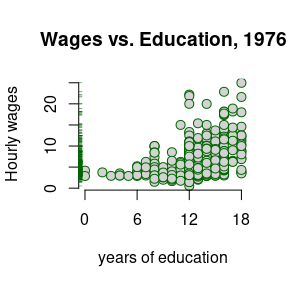It appears that on average, more years of education, leads to higher wages.

The example in the text investigates what the percentage change between wages and education might be. So, we must use the $$log($$wage$$)$$.

Build a linear model to estimate the relationship between the log of wage (lwage) and education (educ).

$\widehat{log(wage)} = \beta_0 + \beta_1educ$

log_wage_model <- lm(lwage ~ educ, data = wage1)

Print the summary of the results.

summary(log_wage_model)
 Dependent variable: lwage educ 0.08274*** (0.00757) Constant 0.58377*** (0.09734) Observations 526 R2 0.18581 Adjusted R2 0.18425 Residual Std. Error 0.48008 (df = 524) F Statistic 119.58160*** (df = 1; 524) Note: p<0.1; p<0.05; p<0.01

Plot the $$log($$wage$$)$$ vs educ, adding a line representing the least squares fit.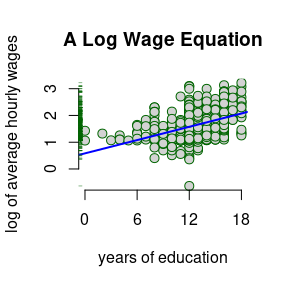## Chapter 3: Multiple Regression Analysis: Estimation

### Example 3.2: Hourly Wage Equation

Check the documentation for variable information

?wage1

$$lwage$$: log of the average hourly earnings

$$educ$$: years of education

$$exper$$: years of potential experience

$$tenutre$$: years with current employer

Plot the variables against lwage and compare their distributions and slope ($$\beta$$) of the simple regression lines.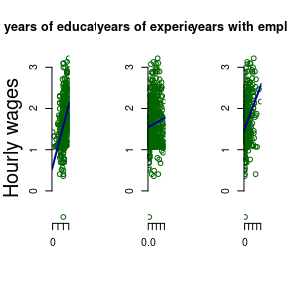Estimate the model regressing educ, exper, and tenure against log(wage).

$\widehat{log(wage)} = \beta_0 + \beta_1educ + \beta_3exper + \beta_4tenure$

hourly_wage_model <- lm(lwage ~ educ + exper + tenure, data = wage1)

Print the estimated model coefficients:

coefficients(hourly_wage_model)
Coefficients
(Intercept) 0.2844
educ 0.0920
exper 0.0041
tenure 0.0221

Plot the coefficients, representing percentage impact of each variable on $$log($$wage$$)$$ for a quick comparison.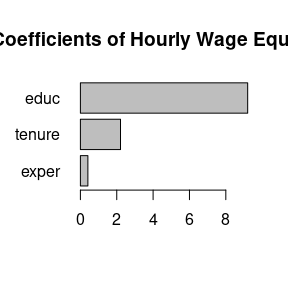## Chapter 4: Multiple Regression Analysis: Inference

### Example 4.1 Hourly Wage Equation

Using the same model estimated in example: 3.2, examine and compare the standard errors associated with each coefficient. Like the textbook, these are contained in parenthesis next to each associated coefficient.

summary(hourly_wage_model)
 Dependent variable: lwage educ 0.09203*** (0.00733) exper 0.00412** (0.00172) tenure 0.02207*** (0.00309) Constant 0.28436*** (0.10419) Observations 526 R2 0.31601 Adjusted R2 0.31208 Residual Std. Error 0.44086 (df = 522) F Statistic 80.39092*** (df = 3; 522) Note: p<0.1; p<0.05; p<0.01

For the years of experience variable, or exper, use coefficient and Standard Error to compute the $$t$$ statistic:

$t_{exper} = \frac{0.004121}{0.001723} = 2.391$

Fortunately, R includes $$t$$ statistics in the summary of model diagnostics.

summary(hourly_wage_model)$coefficients Estimate Std. Error t value Pr(>|t|) (Intercept) 0.28436 0.10419 2.72923 0.00656 educ 0.09203 0.00733 12.55525 0.00000 exper 0.00412 0.00172 2.39144 0.01714 tenure 0.02207 0.00309 7.13307 0.00000 Plot the $$t$$ statistics for a visual comparison: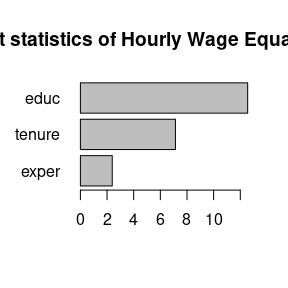### Example 4.7 Effect of Job Training on Firm Scrap Rates Load the jtrain data set. data("jtrain") ?jtrain From H. Holzer, R. Block, M. Cheatham, and J. Knott (1993), Are Training Subsidies Effective? The Michigan Experience, Industrial and Labor Relations Review 46, 625-636. The authors kindly provided the data. $$year:$$ 1987, 1988, or 1989 $$union:$$ =1 if unionized $$lscrap:$$ Log(scrap rate per 100 items) $$hrsemp:$$ (total hours training) / (total employees trained) $$lsales:$$ Log(annual sales,$)

$$lemploy:$$ Log(umber of employees at plant)

First, use the subset function and it’s argument by the same name to return observations which occurred in 1987 and are not union. At the same time, use the select argument to return only the variables of interest for this problem.

jtrain_subset <- subset(jtrain, subset = (year == 1987 & union == 0),
select = c(year, union, lscrap, hrsemp, lsales, lemploy))

Next, test for missing values. One can “eyeball” these with R Studio’s View function, but a more precise approach combines the sum and is.na functions to return the total number of observations equal to NA.

sum(is.na(jtrain_subset))
##  156

While R’s lm function will automatically remove missing NA values, eliminating these manually will produce more clearly proportioned graphs for exploratory analysis. Call the na.omit function to remove all missing values and assign the new data.frame object the name jtrain_clean.

jtrain_clean <- na.omit(jtrain_subset)

Use jtrain_clean to plot the variables of interest against lscrap. Visually observe the respective distributions for each variable, and compare the slope ($$\beta$$) of the simple regression lines.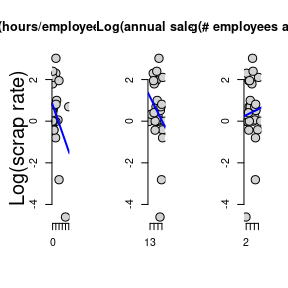Now create the linear model regressing hrsemp(total hours training/total employees trained), lsales(log of annual sales), and lemploy(the log of the number of the employees), against lscrap(the log of the scrape rate).

$lscrap = \alpha + \beta_1 hrsemp + \beta_2 lsales + \beta_3 lemploy$

linear_model <- lm(lscrap ~ hrsemp + lsales + lemploy, data = jtrain_clean)

Finally, print the complete summary diagnostics of the model.

summary(linear_model)
 Dependent variable: lscrap hrsemp -0.02927 (0.02280) lsales -0.96203** (0.45252) lemploy 0.76147* (0.40743) Constant 12.45837** (5.68677) Observations 29 R2 0.26243 Adjusted R2 0.17392 Residual Std. Error 1.37604 (df = 25) F Statistic 2.96504* (df = 3; 25) Note: p<0.1; p<0.05; p<0.01

## Chapter 5: Multiple Regression Analysis: OLS Asymptotics

### Example 5.1: Housing Prices and Distance From an Incinerator

Load the hprice3 data set.

data("hprice3")

$$lprice:$$ Log(selling price)

$$ldist:$$ Log(distance from house to incinerator, feet)

$$larea:$$ Log(square footage of house)

Graph the prices of housing against distance from an incinerator: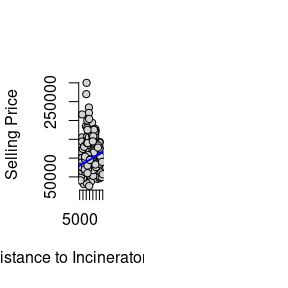Next, model the $$log($$price$$)$$ against the $$log($$dist$$)$$ to estimate the percentage relationship between the two.

$price = \alpha + \beta_1 dist$

price_dist_model <- lm(lprice ~ ldist, data = hprice3)

Create another model that controls for “quality” variables, such as square footage area per house.

$price = \alpha + \beta_1 dist + \beta_2 area$

price_area_model <- lm(lprice ~ ldist + larea, data = hprice3)

Compare the coefficients of both models. Notice that adding area improves the quality of the model, but also reduces the coefficient size of dist.

summary(price_dist_model)
summary(price_area_model)
 Dependent variable: lprice (1) (2) ldist 0.31722*** (0.04811) 0.19623*** (0.03816) larea 0.78368*** (0.05358) Constant 8.25750*** (0.47383) 3.49394*** (0.49065) Observations 321 321 R2 0.11994 0.47385 Adjusted R2 0.11718 0.47054 Residual Std. Error 0.41170 (df = 319) 0.31883 (df = 318) F Statistic 43.47673*** (df = 1; 319) 143.19470*** (df = 2; 318) Note: p<0.1; p<0.05; p<0.01

Graphing illustrates the larger coefficient for area.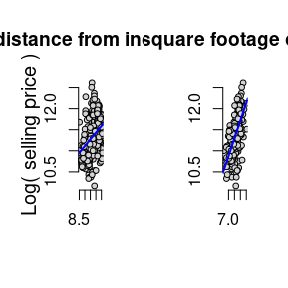## Chapter 6: Multiple Regression: Further Issues

### Example 6.1: Effects of Pollution on Housing Prices, standardized.

Load the hprice2 data and view the documentation.

data("hprice2")
?hprice2

Data from Hedonic Housing Prices and the Demand for Clean Air, by Harrison, D. and D.L.Rubinfeld, Journal of Environmental Economics and Management 5, 81-102. Diego Garcia, a former Ph.D. student in economics at MIT, kindly provided these data, which he obtained from the book Regression Diagnostics: Identifying Influential Data and Sources of Collinearity, by D.A. Belsey, E. Kuh, and R. Welsch, 1990. New York: Wiley.

$$price$$: median housing price.

$$nox$$: Nitrous Oxide concentration; parts per million.

$$crime$$: number of reported crimes per capita.

$$rooms$$: average number of rooms in houses in the community.

$$dist$$: weighted distance of the community to 5 employment centers.

$$stratio$$: average student-teacher ratio of schools in the community.

$price = \beta_0 + \beta_1nox + \beta_2crime + \beta_3rooms + \beta_4dist + \beta_5stratio + \mu$

Estimate the usual lm model.

housing_level <- lm(price ~ nox + crime + rooms + dist + stratio, data = hprice2)

Estimate the same model, but standardized coefficients by wrapping each variable with R’s scale function:

$\widehat{zprice} = \beta_1znox + \beta_2zcrime + \beta_3zrooms + \beta_4zdist + \beta_5zstratio$

housing_standardized <- lm(scale(price) ~ 0 + scale(nox) + scale(crime) + scale(rooms) + scale(dist) + scale(stratio), data = hprice2)

Compare results, and observe

summary(housing_level)
summary(housing_standardized)
 Dependent variable: price scale(price) (1) (2) nox -2,706.43300*** (354.08690) crime -153.60100*** (32.92883) rooms 6,735.49800*** (393.60370) dist -1,026.80600*** (188.10790) stratio -1,149.20400*** (127.42870) scale(nox) -0.34045*** (0.04450) scale(crime) -0.14328*** (0.03069) scale(rooms) 0.51389*** (0.03000) scale(dist) -0.23484*** (0.04298) scale(stratio) -0.27028*** (0.02994) Constant 20,871.13000*** (5,054.59900) Observations 506 506 R2 0.63567 0.63567 Adjusted R2 0.63202 0.63203 Residual Std. Error 5,586.19800 (df = 500) 0.60601 (df = 501) F Statistic 174.47330*** (df = 5; 500) 174.82220*** (df = 5; 501) Note: p<0.1; p<0.05; p<0.01

### Example 6.2: Effects of Pollution on Housing Prices, Quadratic Interactive Term

Modify the housing model from example 4.5, adding a quadratic term in rooms:

$log(price) = \beta_0 + \beta_1log(nox) + \beta_2log(dist) + \beta_3rooms + \beta_4rooms^2 + \beta_5stratio + \mu$

housing_model_4.5 <- lm(lprice ~ lnox + log(dist) + rooms + stratio, data = hprice2)

housing_model_6.2 <- lm(lprice ~ lnox + log(dist) + rooms + I(rooms^2) + stratio,
data = hprice2)

Compare the results with the model from example 6.1.

summary(housing_model_4.5)
summary(housing_model_6.2)
 Dependent variable: lprice (1) (2) lnox -0.95354*** (0.11674) -0.90168*** (0.11469) log(dist) -0.13434*** (0.04310) -0.08678** (0.04328) rooms 0.25453*** (0.01853) -0.54511*** (0.16545) I(rooms2) 0.06226*** (0.01280) stratio -0.05245*** (0.00590) -0.04759*** (0.00585) Constant 11.08386*** (0.31811) 13.38548*** (0.56647) Observations 506 506 R2 0.58403 0.60281 Adjusted R2 0.58071 0.59884 Residual Std. Error 0.26500 (df = 501) 0.25921 (df = 500) F Statistic 175.85520*** (df = 4; 501) 151.77040*** (df = 5; 500) Note: p<0.1; p<0.05; p<0.01

Estimate the minimum turning point at which the rooms interactive term changes from negative to positive.

$x = \frac{\hat{\beta_1}}{2\hat{\beta_2}}$

beta_1 <- summary(housing_model_6.2)$coefficients["rooms",1] beta_2 <- summary(housing_model_6.2)$coefficients["I(rooms^2)",1]
turning_point <- abs(beta_1 / (2*beta_2))

print(turning_point)
##  4.37763

Compute the percent change across a range of average rooms. Include the smallest, turning point, and largest.

Rooms <- c(min(hprice2$rooms), 4, turning_point, 5, 5.5, 6.45, 7.5, max(hprice2$rooms))
Percent.Change <- 100*(beta_1 + 2*beta_2*Rooms)

kable(data.frame(Rooms, Percent.Change))
Rooms Percent.Change
3.56000 -10.181324
4.00000 -4.702338
4.37763 0.000000
5.00000 7.749903
5.50000 13.976023
6.45000 25.805651
7.50000 38.880503
8.78000 54.819367

Graph the log of the selling price against the number of rooms. Superimpose a simple model as well as a quadratic model and examine the difference.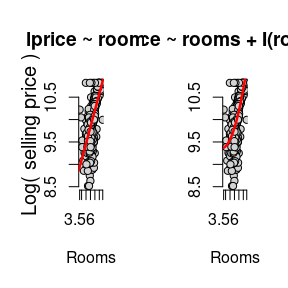## Chapter 7: Multiple Regression Analysis with Qualitative Information

### Example 7.4: Housing Price Regression, Qualitative Binary variable

This time, use the hrprice1 data.

data("hprice1")
?hprice1

Data collected from the real estate pages of the Boston Globe during 1990. These are homes that sold in the Boston, MA area.

$$lprice:$$ Log(house price, $1000s) $$llotsize:$$ Log(size of lot in square feet) $$lsqrft:$$ Log(size of house in square feet) $$bdrms:$$ number of bdrms $$colonial:$$ =1 if home is colonial style $\widehat{log(price)} = \beta_0 + \beta_1log(lotsize) + \beta_2log(sqrft) + \beta_3bdrms + \beta_4colonial$ Estimate the coefficients of the above linear model on the hprice data set. housing_qualitative <- lm(lprice ~ llotsize + lsqrft + bdrms + colonial, data = hprice1) summary(housing_qualitative)  Dependent variable: lprice llotsize 0.16782*** (0.03818) lsqrft 0.70719*** (0.09280) bdrms 0.02683 (0.02872) colonial 0.05380 (0.04477) Constant -1.34959** (0.65104) Observations 88 R2 0.64907 Adjusted R2 0.63216 Residual Std. Error 0.18412 (df = 83) F Statistic 38.37846*** (df = 4; 83) Note: p<0.1; p<0.05; p<0.01 ## Chapter 8: Heteroskedasticity ### Example 8.9: Determinants of Personal Computer Ownership $\widehat{PC} = \beta_0 + \beta_1hsGPA + \beta_2ACT + \beta_3parcoll + \beta_4colonial$ Christopher Lemmon, a former MSU undergraduate, collected these data from a survey he took of MSU students in Fall 1994. Load gpa1 and create a new variable combining the fathcoll and mothcoll, into parcoll. This new column indicates if either parent went to college. data("gpa1") gpa1$parcoll <- as.integer(gpa1$fathcoll==1 | gpa1$mothcoll)

GPA_OLS <- lm(PC ~ hsGPA + ACT + parcoll, data = gpa1)

Calculate the weights and then pass them to the weights argument.

weights <- GPA_OLS$fitted.values * (1-GPA_OLS$fitted.values)

GPA_WLS <- lm(PC ~ hsGPA + ACT + parcoll, data = gpa1, weights = 1/weights)

Compare the OLS and WLS model in the table below:

 Dependent variable: PC (1) (2) hsGPA 0.06539 (0.13726) 0.03270 (0.12988) ACT 0.00056 (0.01550) 0.00427 (0.01545) parcoll 0.22105** (0.09296) 0.21519** (0.08629) Constant -0.00043 (0.49054) 0.02621 (0.47665) Observations 141 141 R2 0.04153 0.04644 Adjusted R2 0.02054 0.02556 Residual Std. Error (df = 137) 0.48599 1.01624 F Statistic (df = 3; 137) 1.97851 2.22404* Note: p<0.1; p<0.05; p<0.01

## Chapter 9: More on Specification and Data Issues

### Example 9.8: R&D Intensity and Firm Size

$rdintens = \beta_0 + \beta_1sales + \beta_2profmarg + \mu$

From Businessweek R&D Scoreboard, October 25, 1991. Load the data and estimate the model.

data("rdchem")

all_rdchem <- lm(rdintens ~ sales + profmarg, data = rdchem)

Plotting the data reveals the outlier on the far right of the plot, which will skew the results of our model.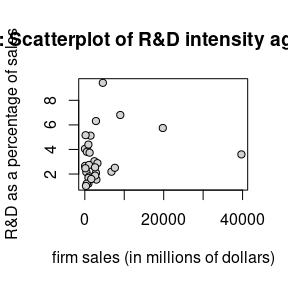So, we can estimate the model without that data point to gain a better understanding of how sales and profmarg describe rdintens for most firms. We can use the subset argument of the linear model function to indicate that we only want to estimate the model using data that is less than the highest sales.

smallest_rdchem <- lm(rdintens ~ sales + profmarg, data = rdchem,
subset = (sales < max(sales)))

The table below compares the results of both models side by side. By removing the outlier firm, $$sales$$ become a more significant determination of R&D expenditures.

 Dependent variable: rdintens (1) (2) sales 0.00005 (0.00004) 0.00019** (0.00008) profmarg 0.04462 (0.04618) 0.04784 (0.04448) Constant 2.62526*** (0.58553) 2.29685*** (0.59180) Observations 32 31 R2 0.07612 0.17281 Adjusted R2 0.01240 0.11372 Residual Std. Error 1.86205 (df = 29) 1.79218 (df = 28) F Statistic 1.19465 (df = 2; 29) 2.92476* (df = 2; 28) Note: p<0.1; p<0.05; p<0.01

## Chapter 10: Basic Regression Analysis with Time Series Data

### Example 10.2: Effects of Inflation and Deficits on Interest Rates

$\widehat{i3} = \beta_0 + \beta_1inf_t + \beta_2def_t$ Data from the Economic Report of the President, 2004, Tables B-64, B-73, and B-79.

data("intdef")

tbill_model <- lm(i3 ~ inf + def, data = intdef)
 Dependent variable: i3 inf 0.60587*** (0.08213) def 0.51306*** (0.11838) Constant 1.73327*** (0.43197) Observations 56 R2 0.60207 Adjusted R2 0.58705 Residual Std. Error 1.84316 (df = 53) F Statistic 40.09424*** (df = 2; 53) Note: p<0.1; p<0.05; p<0.01

### Example 10.11: Seasonal Effects of Antidumping Filings

C.M. Krupp and P.S. Pollard (1999), Market Responses to Antidumpting Laws: Some Evidence from the U.S. Chemical Industry, Canadian Journal of Economics 29, 199-227. Dr. Krupp kindly provided the data. They are monthly data covering February 1978 through December 1988.

data("barium")
barium_imports <- lm(lchnimp ~ lchempi + lgas + lrtwex + befile6 + affile6 + afdec6, data = barium)

Estimate a new model, barium_seasonal which accounts for seasonality by adding dummy variables contained in the data.

barium_seasonal <- lm(lchnimp ~ lchempi + lgas + lrtwex + befile6 + affile6 + afdec6 + feb + mar + apr + may + jun + jul + aug + sep + oct + nov + dec, data = barium)

Compare both models:

 Dependent variable: lchnimp (1) (2) lchempi 3.11719*** (0.47920) 3.26506*** (0.49293) lgas 0.19635 (0.90662) -1.27812 (1.38901) lrtwex 0.98302** (0.40015) 0.66305 (0.47130) befile6 0.05957 (0.26097) 0.13970 (0.26681) affile6 -0.03241 (0.26430) 0.01263 (0.27869) afdec6 -0.56524* (0.28584) -0.52130* (0.30195) feb -0.41771 (0.30444) mar 0.05905 (0.26473) apr -0.45148* (0.26839) may 0.03331 (0.26924) jun -0.20633 (0.26925) jul 0.00384 (0.27877) aug -0.15707 (0.27799) sep -0.13416 (0.26766) oct 0.05169 (0.26685) nov -0.24626 (0.26283) dec 0.13284 (0.27142) Constant -17.80300 (21.04537) 16.77877 (32.42865) Observations 131 131 R2 0.30486 0.35833 Adjusted R2 0.27123 0.26179 Residual Std. Error 0.59735 (df = 124) 0.60121 (df = 113) F Statistic 9.06365*** (df = 6; 124) 3.71190*** (df = 17; 113) Note: p<0.1; p<0.05; p<0.01

Now, compute the anova between the two models.

barium_anova <- anova(barium_imports, barium_seasonal)
 Statistic N Mean St. Dev. Min Pctl(25) Pctl(75) Max Res.Df 2 118.50000 7.77817 113 115.8 121.2 124 RSS 2 42.54549 2.40642 40.84390 41.69469 43.39629 44.24709 Df 1 11.00000 11.00000 11.00000 11.00000 11.00000 Sum of Sq 1 3.40319 3.40319 3.40319 3.40319 3.40319 F 1 0.85594 0.85594 0.85594 0.85594 0.85594 Pr(> F) 1 0.58520 0.58520 0.58520 0.58520 0.58520

## Chapter 11: Further Issues in Using OLS with with Time Series Data

### Example 11.7: Wages and Productivity

$\widehat{log(hrwage_t)} = \beta_0 + \beta_1log(outphr_t) + \beta_2t + \mu_t$ Data from the Economic Report of the President, 1989, Table B-47. The data are for the non-farm business sector.

data("earns")

wage_time <- lm(lhrwage ~ loutphr + t, data = earns)
wage_diff <- lm(diff(lhrwage) ~ diff(loutphr), data = earns)
 Dependent variable: lhrwage diff(lhrwage) (1) (2) loutphr 1.63964*** (0.09335) t -0.01823*** (0.00175) diff(loutphr) 0.80932*** (0.17345) Constant -5.32845*** (0.37445) -0.00366 (0.00422) Observations 41 40 R2 0.97122 0.36424 Adjusted R2 0.96971 0.34750 Residual Std. Error (df = 38) 0.02854 0.01695 F Statistic 641.22430*** (df = 2; 38) 21.77054*** (df = 1; 38) Note: p<0.1; p<0.05; p<0.01

## Chapter 12: Serial Correlation and Heteroskedasticiy in Time Series Regressions

### Example 12.4: Prais-Winsten Estimation in the Event Study

data("barium")

barium_model <- lm(lchnimp ~ lchempi + lgas + lrtwex + befile6 + affile6 + afdec6,
data = barium)

Load the prais package, use the prais_winsten function to estimate.

library(prais)
barium_prais_winsten <- prais_winsten(lchnimp ~ lchempi + lgas + lrtwex + befile6 + affile6 + afdec6, data = barium)
## Iteration 0: rho = 0
## Iteration 1: rho = 0.2708
## Iteration 2: rho = 0.291
## Iteration 3: rho = 0.293
## Iteration 4: rho = 0.2932
## Iteration 5: rho = 0.2932
## Iteration 6: rho = 0.2932
## Iteration 7: rho = 0.2932
 Dependent variable: lchnimp lchempi 3.11719*** (0.47920) lgas 0.19635 (0.90662) lrtwex 0.98302** (0.40015) befile6 0.05957 (0.26097) affile6 -0.03241 (0.26430) afdec6 -0.56524* (0.28584) Constant -17.80300 (21.04537) Observations 131 R2 0.30486 Adjusted R2 0.27123 Residual Std. Error 0.59735 (df = 124) F Statistic 9.06365*** (df = 6; 124) Note: p<0.1; p<0.05; p<0.01
barium_prais_winsten
##
## Call:
## prais_winsten(formula = lchnimp ~ lchempi + lgas + lrtwex + befile6 +
##     affile6 + afdec6, data = barium)
##
## Coefficients:
## (Intercept)      lchempi         lgas       lrtwex      befile6      affile6
##   -37.07770      2.94095      1.04638      1.13279     -0.01648     -0.03316
##      afdec6
##    -0.57681
##
## AR(1) coefficient rho: 0.2932

### Example 12.8: Heteroskedasticity and the Efficient Markets Hypothesis

These are Wednesday closing prices of value-weighted NYSE average, available in many publications. Wooldridge does not recall the particular source used when he collected these data at MIT, but notes probably the easiest way to get similar data is to go to the NYSE web site, www.nyse.com.

$return_t = \beta_0 + \beta_1return_{t-1} + \mu_t$

data("nyse")
?nyse 
return_AR1 <-lm(return ~ return_1, data = nyse)

$\hat{\mu^2_t} = \beta_0 + \beta_1return_{t-1} + residual_t$

return_mu <- residuals(return_AR1)
mu2_hat_model <- lm(return_mu^2 ~ return_1, data = return_AR1\$model)
 Dependent variable: return return_mu2 (1) (2) return_1 0.05890 (0.03802) -1.10413*** (0.20140) Constant 0.17963** (0.08074) 4.65650*** (0.42768) Observations 689 689 R2 0.00348 0.04191 Adjusted R2 0.00203 0.04052 Residual Std. Error (df = 687) 2.11040 11.17847 F Statistic (df = 1; 687) 2.39946 30.05460*** Note: p<0.1; p<0.05; p<0.01

### Example 12.9: ARCH in Stock Returns

$\hat{\mu^2_t} = \beta_0 + \hat{\mu^2_{t-1}} + residual_t$

We still have return_mu in the working environment so we can use it to create $$\hat{\mu^2_t}$$, (mu2_hat) and $$\hat{\mu^2_{t-1}}$$ (mu2_hat_1). Notice the use R’s matrix subset operations to perform the lag operation. We drop the first observation of mu2_hat and squared the results. Next, we remove the last observation of mu2_hat_1 using the subtraction operator combined with a call to the NROW function on return_mu. Now, both contain $$688$$ observations and we can estimate a standard linear model.

mu2_hat  <- return_mu[-1]^2
mu2_hat_1 <- return_mu[-NROW(return_mu)]^2
arch_model <- lm(mu2_hat ~ mu2_hat_1)
 Dependent variable: mu2_hat mu2_hat_1 0.33706*** (0.03595) Constant 2.94743*** (0.44023) Observations 688 R2 0.11361 Adjusted R2 0.11231 Residual Std. Error 10.75907 (df = 686) F Statistic 87.92263*** (df = 1; 686) Note: p<0.1; p<0.05; p<0.01

## Chapter 13: Pooling Cross Sections across Time: Simple Panel Data Methods

### Example 13.7: Effect of Drunk Driving Laws on Traffic Fatalities

Wooldridge collected these data from two sources, the 1992 Statistical Abstract of the United States (Tables 1009, 1012) and A Digest of State Alcohol-Highway Safety Related Legislation, 1985 and 1990, published by the U.S. National Highway Traffic Safety Administration. $\widehat{\Delta{dthrte}} = \beta_0 + \Delta{open} + \Delta{admin}$

data("traffic1")
?traffic1
DD_model <- lm(cdthrte ~ copen + cadmn, data = traffic1)
 Dependent variable: cdthrte copen -0.41968** (0.20559) cadmn -0.15060 (0.11682) Constant -0.49679*** (0.05243) Observations 51 R2 0.11867 Adjusted R2 0.08194 Residual Std. Error 0.34350 (df = 48) F Statistic 3.23144** (df = 2; 48) Note: p<0.1; p<0.05; p<0.01

## Chapter 14: Advanced Panel Data Methods

### Example 14.1: Effect of Job Training on Firm Scrap Rates

In this section, we will estimate a linear panel model using the plm function from the plm: Linear Models for Panel Data package. See the bibliography for more information.

library(plm)
data("jtrain")
scrap_panel <- plm(lscrap ~ d88 + d89 + grant + grant_1, data = jtrain,
index = c('fcode','year'), model = 'within', effect ='individual')
 Dependent variable: lscrap d88 -0.08022 (0.10948) d89 -0.24720* (0.13322) grant -0.25231* (0.15063) grant_1 -0.42159** (0.21020) Observations 162 R2 0.20105 Adjusted R2 -0.23684 F Statistic 6.54259*** (df = 4; 104) Note: p<0.1; p<0.05; p<0.01

## Chapter 15: Instrumental Variables Estimation and Two Stage Least Squares

### Example 15.1: Estimating the Return to Education for Married Women

T.A. Mroz (1987), The Sensitivity of an Empirical Model of Married Women’s Hours of Work to Economic and Statistical Assumptions, Econometrica 55, 765-799. Professor Ernst R. Berndt, of MIT, kindly provided the data, which he obtained from Professor Mroz.

$log(wage) = \beta_0 + \beta_1educ + \mu$

data("mroz")
?mroz
wage_educ_model <- lm(lwage ~ educ, data = mroz)

$\widehat{educ} = \beta_0 + \beta_1fatheduc$

We run the typical linear model, but notice the use of the subset argument. inlf is a binary variable in which a value of 1 means they are “In the Labor Force”. By sub-setting the mroz data.frame by observations in which inlf==1, only working women will be in the sample.

fatheduc_model <- lm(educ ~ fatheduc, data = mroz, subset = (inlf==1))

In this section, we will perform an Instrumental-Variable Regression, using the ivreg function in the AER (Applied Econometrics with R) package. See the bibliography for more information.

library(AER)
wage_educ_IV <- ivreg(lwage ~ educ | fatheduc, data = mroz)
 Dependent variable: lwage educ lwage OLS OLS instrumental variable (1) (2) (3) educ 0.10865*** (0.01440) 0.05917* (0.03514) fatheduc 0.26944*** (0.02859) Constant -0.18520 (0.18523) 10.23705*** (0.27594) 0.44110 (0.44610) Observations 428 428 428 R2 0.11788 0.17256 0.09344 Adjusted R2 0.11581 0.17062 0.09131 Residual Std. Error (df = 426) 0.68003 2.08130 0.68939 F Statistic (df = 1; 426) 56.92892*** 88.84076*** Note: p<0.1; p<0.05; p<0.01

### Example 15.2: Estimating the Return to Education for Men

Data from M. Blackburn and D. Neumark (1992), Unobserved Ability, Efficiency Wages, and Interindustry Wage Differentials, Quarterly Journal of Economics 107, 1421-1436. Professor Neumark kindly provided the data, of which Wooldridge uses the data for 1980.

$\widehat{educ} = \beta_0 + sibs$

data("wage2")
?wage2
educ_sibs_model <- lm(educ ~ sibs, data = wage2)

$\widehat{log(wage)} = \beta_0 + educ$

Again, estimate the model using the ivreg function in the AER (Applied Econometrics with R) package.

library(AER)
educ_sibs_IV <- ivreg(lwage ~ educ | sibs, data = wage2)
 Dependent variable: educ lwage OLS instrumental variable (1) (2) (3) sibs -0.22792*** (0.03028) educ 0.12243*** (0.02635) 0.05917* (0.03514) Constant 14.13879*** (0.11314) 5.13003*** (0.35517) 0.44110 (0.44610) Observations 935 935 428 R2 0.05726 -0.00917 0.09344 Adjusted R2 0.05625 -0.01026 0.09131 Residual Std. Error 2.13398 (df = 933) 0.42330 (df = 933) 0.68939 (df = 426) F Statistic 56.66715*** (df = 1; 933) Note: p<0.1; p<0.05; p<0.01

### Example 15.5: Return to Education for Working Women

$\widehat{log(wage)} = \beta_0 + \beta_1educ + \beta_2exper + \beta_3exper^2$

Use the ivreg function in the AER (Applied Econometrics with R) package to estimate.

data("mroz")
wage_educ_exper_IV <- ivreg(lwage ~ educ + exper + expersq | exper + expersq + motheduc + fatheduc, data = mroz)
 Dependent variable: lwage educ 0.06140* (0.03144) exper 0.04417*** (0.01343) expersq -0.00090** (0.00040) Constant 0.04810 (0.40033) Observations 428 R2 0.13571 Adjusted R2 0.12959 Residual Std. Error 0.67471 (df = 424) Note: p<0.1; p<0.05; p<0.01

## Chapter 16: Simultaneous Equations Models

### Example 16.4: INFLATION AND OPENNESS

Data from D. Romer (1993), Openness and Inflation: Theory and Evidence, Quarterly Journal of Economics 108, 869-903. The data are included in the article.

$inf = \beta_{10} + \alpha_1open + \beta_{11}log(pcinc) + \mu_1$ $open = \beta_{20} + \alpha_2inf + \beta_{21}log(pcinc) + \beta_{22}log(land) + \mu_2$

### Example 16.6: INFLATION AND OPENNESS

$\widehat{open} = \beta_0 + \beta_{1}log(pcinc) + \beta_{2}log(land)$

data("openness")
?openness
open_model <-lm(open ~ lpcinc + lland, data = openness)

$\widehat{inf} = \beta_0 + \beta_{1}open + \beta_{2}log(pcinc)$

Use the ivreg function in the AER (Applied Econometrics with R) package to estimate.

library(AER)
inflation_IV <- ivreg(inf ~ open + lpcinc | lpcinc + lland, data = openness)
 Dependent variable: open inf OLS instrumental variable (1) (2) open -0.33749** (0.14412) lpcinc 0.54648 (1.49324) 0.37582 (2.01508) lland -7.56710*** (0.81422) Constant 117.08450*** (15.84830) 26.89934* (15.40120) Observations 114 114 R2 0.44867 0.03088 Adjusted R2 0.43873 0.01341 Residual Std. Error (df = 111) 17.79559 23.83581 F Statistic 45.16537*** (df = 2; 111) Note: p<0.1; p<0.05; p<0.01

## Chapter 18: Advanced Time Series Topics

### Example 18.8: FORECASTING THE U.S. UNEMPLOYMENT RATE

Data from Economic Report of the President, 2004, Tables B-42 and B-64.

data("phillips")
?phillips

$\widehat{unemp_t} = \beta_0 + \beta_1unem_{t-1}$

Estimate the linear model in the usual way and note the use of the subset argument to define data equal to and before the year 1996.

phillips_train <- subset(phillips, year <= 1996)

unem_AR1 <- lm(unem ~ unem_1, data = phillips_train)

$\widehat{unemp_t} = \beta_0 + \beta_1unem_{t-1} + \beta_2inf_{t-1}$

unem_inf_VAR1 <- lm(unem ~ unem_1 + inf_1, data = phillips_train)
 Dependent variable: unem (1) (2) unem_1 0.73235*** (0.09689) 0.64703*** (0.08381) inf_1 0.18358*** (0.04118) Constant 1.57174*** (0.57712) 1.30380** (0.48969) Observations 48 48 R2 0.55397 0.69059 Adjusted R2 0.54427 0.67684 Residual Std. Error 1.04857 (df = 46) 0.88298 (df = 45) F Statistic 57.13184*** (df = 1; 46) 50.21941*** (df = 2; 45) Note: p<0.1; p<0.05; p<0.01

Now, use the subset argument to create our testing data set containing observation after 1996. Next, pass the both the model object and the test set to the predict function for both models. Finally, cbind or “column bind” both forecasts as well as the year and unemployment rate of the test set.

phillips_test <- subset(phillips, year >= 1997)

AR1_forecast <- predict.lm(unem_AR1, newdata = phillips_test)
VAR1_forecast <- predict.lm(unem_inf_VAR1, newdata = phillips_test)

kable(cbind(phillips_test[ ,c("year", "unem")], AR1_forecast, VAR1_forecast))
year unem AR1_forecast VAR1_forecast
50 1997 4.9 5.526452 5.348468
51 1998 4.5 5.160275 4.896451
52 1999 4.2 4.867333 4.509137
53 2000 4.0 4.647627 4.425175
54 2001 4.8 4.501157 4.516062
55 2002 5.8 5.087040 4.923537
56 2003 6.0 5.819394 5.350271

# Appendix

### Using R for Introductory Econometrics

This is an excellent open source complimentary text to “Introductory Econometrics” by Jeffrey M. Wooldridge and should be your number one resource. This excerpt from the book’s website:

This book introduces the popular, powerful and free programming language and software package R with a focus on the implementation of standard tools and methods used in econometrics. Unlike other books on similar topics, it does not attempt to provide a self-contained discussion of econometric models and methods. Instead, it builds on the excellent and popular textbook “Introductory Econometrics” by Jeffrey M. Wooldridge.

Hess, Florian. Using R for Introductory Econometrics. ISBN: 978-1-523-28513-6, CreateSpace Independent Publishing Platform, 2016, Dusseldorf, Germany.

### Applied Econometrics with R

From the publisher’s website:

This is the first book on applied econometrics using the R system for statistical computing and graphics. It presents hands-on examples for a wide range of econometric models, from classical linear regression models for cross-section, time series or panel data and the common non-linear models of microeconometrics such as logit, probit and tobit models, to recent semiparametric extensions. In addition, it provides a chapter on programming, including simulations, optimization, and an introduction to R tools enabling reproducible econometric research. An R package accompanying this book, AER, is available from the Comprehensive R Archive Network (CRAN) at https://CRAN.R-project.org/package=AER.

Kleiber, Christian and Achim Zeileis. Applied Econometrics with R. ISBN 978-0-387-77316-2, Springer-Verlag, 2008, New York. https://www.springer.com/us/book/9780387773162

## Bibliography

Croissant Y, Millo G (2008). “Panel Data Econometrics in R: The plm Package.” Journal of Statistical Software, 27(2), 1-43. doi: 10.18637/jss.v027.i02 (URL: https://doi.org/10.18637/jss.v027.i02).

Marek Hlavac (2018). stargazer: Well-Formatted Regression and Summary Statistics Tables. R package version 5.2.1. URL: https://CRAN.R-project.org/package=stargazer

R Core Team (2021). R: A language and environment for statistical computing. R Foundation for Statistical Computing, Vienna, Austria. URL https://www.R-project.org/.

Christian Kleiber and Achim Zeileis (2008). Applied Econometrics with R. New York: Springer-Verlag. ISBN 978-0-387-77316-2. URL: https://CRAN.R-project.org/package=AER

Franz X. Mohr (2019). prais: Prais-Winsten Estimator for AR(1) Serial Correlation. R package version 1.1.1. URL: https://CRAN.R-project.org/package=prais

van der Loo M (2020). “A method for deriving information from running R code.” The R Journal, Accepted for publication. <URL: https://arxiv.org/abs/2002.07472>.

Jeffrey M. Wooldridge (2020). Introductory Econometrics: A Modern Approach, 7th edition. ISBN-13: 978-1-337-55886-0. Mason, Ohio :South-Western Cengage Learning.

Yihui Xie (2021). knitr: A General-Purpose Package for Dynamic Report Generation in R. R package version 1.33. https://CRAN.R-project.org/package=knitr